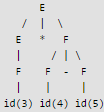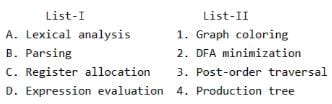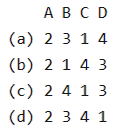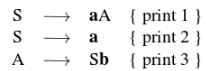Test: Parsing- 2

# Test: Parsing- 2

Test Description

## 20 Questions MCQ Test RRB JE for Computer Science Engineering | Test: Parsing- 2

Test: Parsing- 2 for Computer Science Engineering (CSE) 2022 is part of RRB JE for Computer Science Engineering preparation. The Test: Parsing- 2 questions and answers have been prepared according to the Computer Science Engineering (CSE) exam syllabus.The Test: Parsing- 2 MCQs are made for Computer Science Engineering (CSE) 2022 Exam. Find important definitions, questions, notes, meanings, examples, exercises, MCQs and online tests for Test: Parsing- 2 below.
Solutions of Test: Parsing- 2 questions in English are available as part of our RRB JE for Computer Science Engineering for Computer Science Engineering (CSE) & Test: Parsing- 2 solutions in Hindi for RRB JE for Computer Science Engineering course. Download more important topics, notes, lectures and mock test series for Computer Science Engineering (CSE) Exam by signing up for free. Attempt Test: Parsing- 2 | 20 questions in 60 minutes | Mock test for Computer Science Engineering (CSE) preparation | Free important questions MCQ to study RRB JE for Computer Science Engineering for Computer Science Engineering (CSE) Exam | Download free PDF with solutions
 1 Crore+ students have signed up on EduRev. Have you?
Test: Parsing- 2 - Question 1

### Consider the following expression grammar. The seman­tic rules for expression calculation are stated next to each grammar production. E → number E.val = number. val | E '+' E E(1).val = E(2).val + E(3).val | E '×' E E(1).val = E(2).val × E(3).val   Q. The above grammar and the semantic rules are fed to a yacc tool (which is an LALR (1) parser generator) for parsing and evaluating arithmetic expressions. Which one of the following is true about the action of yacc for the given grammar?

Detailed Solution for Test: Parsing- 2 - Question 1

Background yacc conflict resolution is done using following rules: shift is preferred over reduce while shift/reduce conflict. first reduce is preferred over others while reduce/reduce conflict.   You can answer to this question straightforward by constructing LALR(1) parse table, though its a time taking process. To answer it faster, one can see intuitively that this grammar will have a shift-reduce conflict for sure. In that case, given this is a single choice question, (C) option will be the right answer. Fool-proof explanation would be to generate LALR(1) parse table, which is a lengthy process. Once we have the parse table with us, we can clearly see that i. reduce/reduce conflict will not arise in the above given grammar ii. shift/reduce conflict will be resolved by giving preference to shift, hence making the expression calculator right associative. According to the above conclusions, only correct option seems to be (C).

Test: Parsing- 2 - Question 2

### Consider the following expression grammar. The seman­tic rules for expression calculation are stated next to each grammar production. E → number E.val = number. val | E '+' E E(1).val = E(2).val + E(3).val | E '×' E E(1).val = E(2).val × E(3).val   Q. Assume the conflicts in Part (a) of this question are resolved and an LALR(1) parser is generated for parsing arithmetic expressions as per the given grammar. Consider an expression 3 × 2 + 1. What precedence and associativity properties does the generated parser realize?

Detailed Solution for Test: Parsing- 2 - Question 2

Answer is B as the productions belong to the same non-terminal and since YACC resolves by shift over reduce, the associativity will be right associative.

Test: Parsing- 2 - Question 3

### Which of the following grammar rules violate the requirements of an operator grammar ? P, Q, R are nonterminals, and r, s, t are terminals. 1. P → Q R 2. P → Q s R 3. P → ε 4. P → Q t R r

Test: Parsing- 2 - Question 4

Consider the grammar with the following translation rules and E as the start symbol.

E → E1 # T {E.value = E1.value * T.value}
| T{E.value = T.value}
T → T1 & F {T.value = T1.value + F.value}
| F{T.value = F.value}
F → num {F.value = num.value}

Q. Compute E.value for the root of the parse tree for the expression: 2 # 3 & 5 # 6 & 4.

Test: Parsing- 2 - Question 5

Which of the following suffices to convert an arbitrary CFG to an LL(1) grammar?

Detailed Solution for Test: Parsing- 2 - Question 5

Removing left recursion and factoring the grammar do not suffice to convert an arbitrary CFG to LL(1) grammar.

Test: Parsing- 2 - Question 6

Assume that the SLR parser for a grammar G has n1 states and the LALR parser for G has n2 states. The relationship between n1 and n2 is:

Test: Parsing- 2 - Question 7

In a bottom-up evaluation of a syntax directed definition, inherited attributes can

Detailed Solution for Test: Parsing- 2 - Question 7

A Syntax Directed Definition (SDD) is called S Attributed if it has only synthesized attributes. L-Attributed Definitions contain both synthesized and inherited attributes but do not need to build a dependency graph to evaluate them.

Test: Parsing- 2 - Question 8

Consider the grammar shown below S → i E t S S' | a S' → e S | ε E → b In the predictive parse table. M, of this grammar, the entries M[S', e] and M[S', \$] respectively are

Detailed Solution for Test: Parsing- 2 - Question 8

Here representing the parsing table as M[ X , Y], where X represents rows(Non terminals) and Y represents columns(terminals). Here are the rules to fill the parsing table. For each distinct production rule A->α, of the grammar, we need to apply the given rules:
Rule 1: if A –> α is a production, for each terminal ‘a’ in FIRST(α), add A–>α to M[ A , a]
Rule 2 : if ‘ ε ‘ is in FIRST(α), add A –> α to M [A , b] for each ‘b’ in FOLLOW(A). As Entries have been asked corresponding to Non-Terminal S', hence we only need to consider its productions to get the answer. For S' → eS, according to rule 1, this production rule should be placed at the entry M[ S', FIRST(eS)], and from the given grammar, FIRST(eS) ={e}, hence S'->eS is placed in the parsing table at entry M[S' , e]. Similarly, For S'->ε, as FIRST(ε) = {ε}, hence rule 2 should be applied, therefore, this production rule should be placed in the parsing table at entry M[S',FOLLOW(S')], and FOLLOW(S') = FOLLOW(S) = {e, \$}, hence R->ε is placed at entry M[S', e] and M[S' , \$]. Therefore Answer is option D. Visit the Following links to Learn how to find First and Follow sets.

Test: Parsing- 2 - Question 9

Consider the grammar shown below.

S → C C
C → c C | d

The grammar is

Detailed Solution for Test: Parsing- 2 - Question 9

Since there is no conflict, the grammar is LL(1). We can construct a predictive parse table with no conflicts. This grammar also LR(0), SLR(1), CLR(1) and LALR(1).

Test: Parsing- 2 - Question 10

Consider the translation scheme shown below

S → T R
R → + T {print ('+');} R | ε
T → num {print (num.val);}

Here num is a token that represents an integer and num.val represents the corresponding integer value. For an input string '9 + 5 + 2', this translation scheme will print

Detailed Solution for Test: Parsing- 2 - Question 10

Let us make the parse tree for 9+5+2 in top down manner, left first derivation.

Steps:
1) Exapnd S->TR
2) apply T->Num...
3) apply R -> +T...
4) appy T->Num...
5) apply R-> +T..
6) apply T-> Num..
7) apply R-> epsilon

After printing through the print statement in the parse tree formed you will get the answer as 95+2+

Test: Parsing- 2 - Question 11

Consider the syntax directed definition shown below.

S → id : = E {gen (id.place = E.place;);}
E → E1 + E2 {t = newtemp ( ); gen (t = El.place + E2.place;); E.place = t}
E → id {E.place = id.place;}

Here, gen is a function that generates the output code, and newtemp is a function that returns the name of a new temporary variable on every call. Assume that ti's are the temporary variable names generated by newtemp. For the statement 'X: = Y + Z', the 3-address code sequence generated by this definition is

Detailed Solution for Test: Parsing- 2 - Question 11

It must be B. The production E --> E + E is used only one time and hence only one temporary variable is generated.

Test: Parsing- 2 - Question 12

Which of the following statements is false?

Detailed Solution for Test: Parsing- 2 - Question 12

1. A grammar is ambiguous if there exists a string s such that the grammar has more than one leftmost derivations for s. We could also come up with more than one rightmost derivations for a string to prove the above proposition, but not both of right and leftmost. An unambiguous grammar can have different rightmost and leftmost derivations.
2. LL parser is top-down by nature. Leftmost derivation is, intuitively, expanding or top-down in fashion, hence such convention. Rightmost derivation, on the other hand, seems like a compressing or bottom-up thing.
3. LALR is more powerful than SLR, even when both have the same LR(0) states, due to the fact that SLR checks for lookaheads by looking at FIRST and FOLLOW from the grammar after constructing its parse table and on the other hand, LALR computes lookaheads from the LR(0) states while constructing the parse table, which is a better method.
4. An ambiguous grammar can never be LR(k) for any k, because LR(k) algorithm aren’t designed to handle ambiguous grammars. It would get stuck into undecidability problem, if employed upon an ambiguous grammar, no matter how large the constant k is

Test: Parsing- 2 - Question 13

Which of the following derivations does a top-down parser use while parsing an input string? The input is assumed to be scanned in left to right order.

Test: Parsing- 2 - Question 14

Given the following expression grammar:

E -> E * F | F + E | F
F -> F - F | id

which of the following is true? (GATE CS 2000)

Detailed Solution for Test: Parsing- 2 - Question 14

Let say i/p is 3*4-5 when we draw parse tree according to grammarAs we can see first '- ' will be evaluated then ' * ' is evaluated so ' - ' has higher precedence then *. So correct choice is B See question 1 of

Test: Parsing- 2 - Question 15

Which one of the following is True at any valid state in shift-reduce parsing?

Detailed Solution for Test: Parsing- 2 - Question 15

The prefixes of right sentential forms that can appear on the stack of a shift-reduce parser are called viable prefixes. By definition, a viable prefix is a prefix of a right sentential form that does not continue past the right end of the rightmost handle of that sentential form.

Test: Parsing- 2 - Question 16

In the context of abstract-syntax-tree (AST) and control-flow-graph (CFG), which one of the following is True?

Detailed Solution for Test: Parsing- 2 - Question 16

(A) is false, In CFG (Control Flow Graph), code of N2 may be present before N1 when there is a loop or goto.
(B) is false, CFG (Control Flow Graph) contains cycle when input program has loop.
(C) is true, successors in AST and CFG depedend on input program
(D) is false, a single statement may belong to a block of statements.

Test: Parsing- 2 - Question 17

Match the following:Codes:Detailed Solution for Test: Parsing- 2 - Question 17

Register allocation is a variation of Graph Coloring problem.
Lexical Analysis uses DFA.
Parsing makes production tree Expression evaluation is done using tree traversal

Test: Parsing- 2 - Question 18

Among simple LR (SLR), canonical LR, and look-ahead LR (LALR), which of the following pairs identify the method that is very easy to implement and the method that is the most powerful, in that order?

Detailed Solution for Test: Parsing- 2 - Question 18

SLR parser is a type of LR parser with small parse tables and a relatively simple parser generator algorithm. Canonical LR parser or LR(1) parser is an LR(k) parser for k=1, i.e. with a single lookahead terminal. It can handle all deterministic context-free languages.LALR parser or Look-Ahead LR parser is a simplified version of a canonical LR parser,

Test: Parsing- 2 - Question 19

Consider the following grammar G.

S → F ⎪ H
F → p ⎪ c
H → d ⎪ c

Q. Where S, F and H are non-terminal symbols, p, d and c are terminal symbols. Which of the following statement(s) is/are correct?

S1: LL(1) can parse all strings that are generated using grammar G.
S2: LR(1) can parse all strings that are generated using grammar G.

Detailed Solution for Test: Parsing- 2 - Question 19

The given grammar is ambiguous as there are two possible leftmost derivations for string "c".

First Leftmost Derivation
S → F
F → c

Second Leftmost Derivation
S → H
H → c

An Ambiguous grammar can neither be LL(1) nor LR(1)

Test: Parsing- 2 - Question 20

Consider the following Syntax Directed Translation Scheme (SDTS), with non-terminals {S, A} and terminals {a, b}}.Using the above SDTS, the output printed by a bottom-up parser, for the input aab is

Detailed Solution for Test: Parsing- 2 - Question 20

Bottom up parser builds the parse tree from bottom to up, i.e from the given string to the starting symbol. The given string is aab and starting symbol is S. so the process is to start from aab and reach S. =>aab ( given string) =>aSb (after reduction by S->a, and hence print 2) =>aA (after reduction by A->Sb, and hence print 3) =>S (after reduction by S->aA, and hence print 1) As we reach the starting symbol from the string, the string belongs to the language of the grammar. Another way to do the same thing is :- bottom up parser does the parsing by RMD in reverse. RMD is as follows: =>S => aA (hence, print 1) => aSb (hence, print 3) => aab (hence, print 2) If we take in Reverse it will print : 231

## RRB JE for Computer Science Engineering

3 videos|7 docs|100 tests
 Use Code STAYHOME200 and get INR 200 additional OFF Use Coupon Code
Information about Test: Parsing- 2 Page
In this test you can find the Exam questions for Test: Parsing- 2 solved & explained in the simplest way possible. Besides giving Questions and answers for Test: Parsing- 2, EduRev gives you an ample number of Online tests for practice

## RRB JE for Computer Science Engineering

3 videos|7 docs|100 tests